# Excel 2010: Finding Factorial (FACT Function)

Excel 2010 contains many advance mathematical functions which have been extensively used for different calculations, from a list factorial finder is an important one. Excel FACT function which stands for FACTorial is used for finding out the factorial of the specified number. It require a single argument to be passed, along with this Excel 2010 also include a function which could find out factorial of alternate numbers called FACTDOUBLE.

Open Excel 2010 spreadsheet on which you want to apply FACT functions. For instance we have included a spreadsheet containing fields; S.No, Value, and Factorial.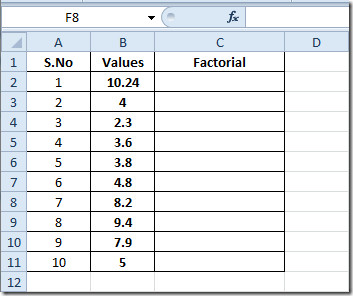Now we need to find out the factorial of data present in Values field, we will be entering the function in the first row of field Factorial.

The syntax of the function is;

=FACT(number)

Single argument number is required, which can either be a number as a direct argument or location of cell where the data value is residing.

We will be write this function as;

=FACT(B2)

B2 is the location of the cell in Values field. It will yield the factorial of the corresponding number, as shown in the screenshot below.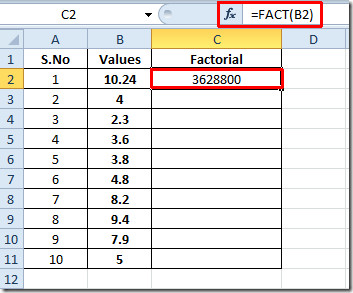Now drag the plus sign at the end of the cell towards the end of the column to apply it over.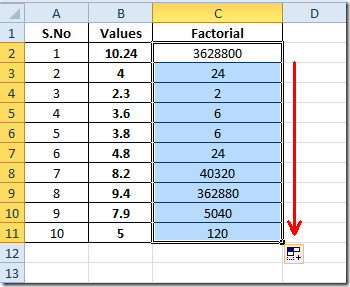If you want to show the factorial of the number directly then enter the value directly as an argument.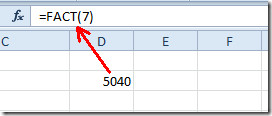For finding out the double factorial, i.e, multiplication with alternate number (6!!=6*4*2), then simply enter;

=FACTDOUBLE(number)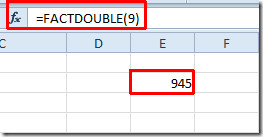You can also check out previously reviewed Excel functions; MAX,MIN, MAXA, MINA and Logical Functions, INFO, SUMSQ, DOLLAR, SUMPRODUCT, SUMIF, COUNTIF, VLOOKUP, HLOOKUP ,PMT, & LEN.

1.kinjal says:
2.csgwga says: